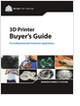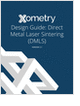Formula HomeFluid TheoryBrief OverviewNavier-StokesBernoulliFluid StaticsGlossaryFlowmetersCalculatorsManometerDraining TankOrifice FlowmeterVenturi FlowmeterPipe FrictionReynolds NumberResourcesBibliography
Selecting the Right 3D Printer

Discover how to choose the right 3D printer for your needs and the key performance attributes to consider.

3D Scanners

A white paper to assist in the evaluation of 3D scanning hardware solutions.

Metal 3D Printing Design Guide

Direct Metal Laser Sintering (DMLS) 3D printing for parts with reduced cost and little waste.

Injection Molding Design Guide

Guide for high quality and cost-effective plastic injection molding.

more free publicationsIntroductionA fluid passing though an orifice constriction will experience a drop in pressure across the orifice. This change can be used to measure the flowrate of the fluid. To calculate the flowrate of a fluid passing through an orifice plate, enter the parameters below. (The default calculation involves air passing through a medium-sized orifice in a 4" pipe, with answers rounded to 3 significant figures.)InputsPipe (inlet) diameter upstream of orifice, Di: m in ft cm Orifice diameter (less than the inlet diameter), Do: m in ft cm Pressure difference across the orifice, Dp: Pa mmHg inH2O inHg ftH2O psi Fluid density, r: kg/m^3 lb/in^3 kg/l Flow Coefficient, Cf:Velocity at the inlet, Vi: 1.76  m/s cm/s in/s ft/s m/s mph Volumetric Flowrate, Q: 13.9  l/s m^3/s l/s ft^3/s in^3/s cm^3/s gal/s gpm pt/s ft^3/min Mass Flowrate: 0.0179  kg/s kg/s slug/s lbm/sEquations used in this CalculatorAs long as the fluid speed is sufficiently subsonic (V < mach 0.3), the incompressible Bernoulli's equation describes the flow reasonably well. Applying this equation to a streamline traveling down the axis of the horizontal tube gives,where location 1 is upstream of the orifice, and location 2 is slightly behind the orifice. It is recommended that location 1 be positioned one pipe diameter upstream of the orifice, and location 2 be positioned one-half pipe diameter downstream of the orifice. Since the pressure at 1 will be higher than the pressure at 2 (for flow moving from 1 to 2), the pressure difference as defined will be a positive quantity. From continuity, the velocities can be replaced by cross-sectional areas of the flow and the volumetric flowrate Q,Solving for the volumetric flowrate Q gives,The above equation applies only to perfectly laminar, inviscid flows. For real flows (such as water or air), viscosity and turbulence are present and act to convert kinetic flow energy into heat. To account for this effect, a discharge coefficient Cd is introduced into the above equation to marginally reduce the flowrate Q,Since the actual flow profile at location 2 downstream of the orifice is quite complex, thereby making the effective value of A2 uncertain, the following substitution introducing a flow coefficient Cf is made,where Ao is the area of the orifice. As a result, the volumetric flowrate Q for real flows is given by the equation,The flow coefficient Cf is found from experiments and is tabulated in reference books; it ranges from 0.6 to 0.9 for most orifices. Since it depends on the orifice and pipe diameters (as well as the Reynolds Number), one will often find Cf tabulated versus the ratio of orifice diameter to inlet diameter, sometimes defined as b,The mass flowrate can be found by multiplying Q with the fluid density,GlossarySelecting the Right 3D Printer

Discover how to choose the right 3D printer for your needs and the key performance attributes to consider.3D Scanners

A white paper to assist in the evaluation of 3D scanning hardware solutions.Metal 3D Printing Design Guide

Direct Metal Laser Sintering (DMLS) 3D printing for parts with reduced cost and little waste.Injection Molding Design Guide

Guide for high quality and cost-effective plastic injection molding.